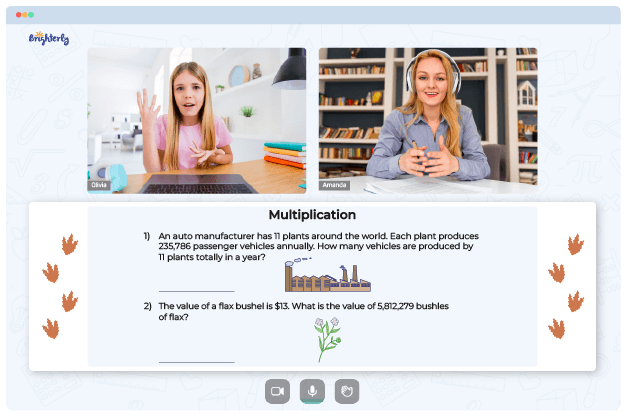4th grade math multiplication worksheets are essential in strengthening kids’ knowledge of math. As multiplication is a complex yet crucial concept, these worksheets simplify the studying process through fun exercises and problems that help kids recognize the questions’ patterns. The design of multiplication 4th grade math worksheets also allows parents to be more hands-on with their children’s math education.

## Free 4th Grade Multiplication Worksheets from Brighterly’s Tutors

Multiplication worksheets for 4th graders’ use are chock-full of practice tests and guidelines to help kids solve even complicated problems. Brighterly builds on children’s foundation in math by designing worksheets that are real teaching aids. Whether you are a tutor, homeschooler, or parent, you will have an excellent time using Brighterly’s math worksheets. These worksheets are designed with precision, and their quality and value are optimal for the users.

As a tutor, you can use these multiplication worksheets for grade 4 as an engaging classroom activity and assist kids in learning in groups or individually. Multiplication worksheets will definitely make your students eager to learn and solve multiplication problems.

Math for Kids

Is Your Child Struggling With Math?
1:1 Online Math TutoringFree multiplication worksheets are also ideal because some families may not afford buying textbooks or printed materials. Their kids shouldn’t miss the worksheets’ benefits like formula retention and pattern recognition. Children will become more accurate as they repeat multiplication questions and problems, improving their precision.

## Printable Multiplication Worksheets for Grade 4 in Pdf

Worksheets are an easy method for kids to learn and practice math. The best part is that you can use them offline by printing and giving them to students in class. So, consider downloading printable multiplication worksheets for grade 4 to help students polish their multiplication skills.

Helping your kid with online math lessons for 4th grade. Expert tutors inspiring kids to love math so they can excel at It.

## Good Times Await Kids with Multiplication Worksheets for Grade 4### Multiplication with Operands Up to 100### Multiply Fractions by Whole Numbers### Multiply Two Numbers Up to 1000### Choose Properties of Multiplication

Using 4th grade multiplication worksheets, students can learn to solve expansion problems, which is handy in almost every career or field they choose later in life. Worksheets also teach kids to multiply numbers in their heads without writing them down or using a multiplication table.

The questions in grade 4 multiplication worksheets range from simple to complex ones. In case of regular practice with multiplication worksheets for 4th grade, students will become better mathematicians. They will also become more effective time and resource managers as worksheets teach children to solve problems faster.

### More Multiplication Worksheets

Need help with Multiplication?• Does your child need extra support with mastering of multiplication?
• Start lessons with an online tutor.

Is your child finding it hard to grasp multiplication? An online tutor could be of assistance.